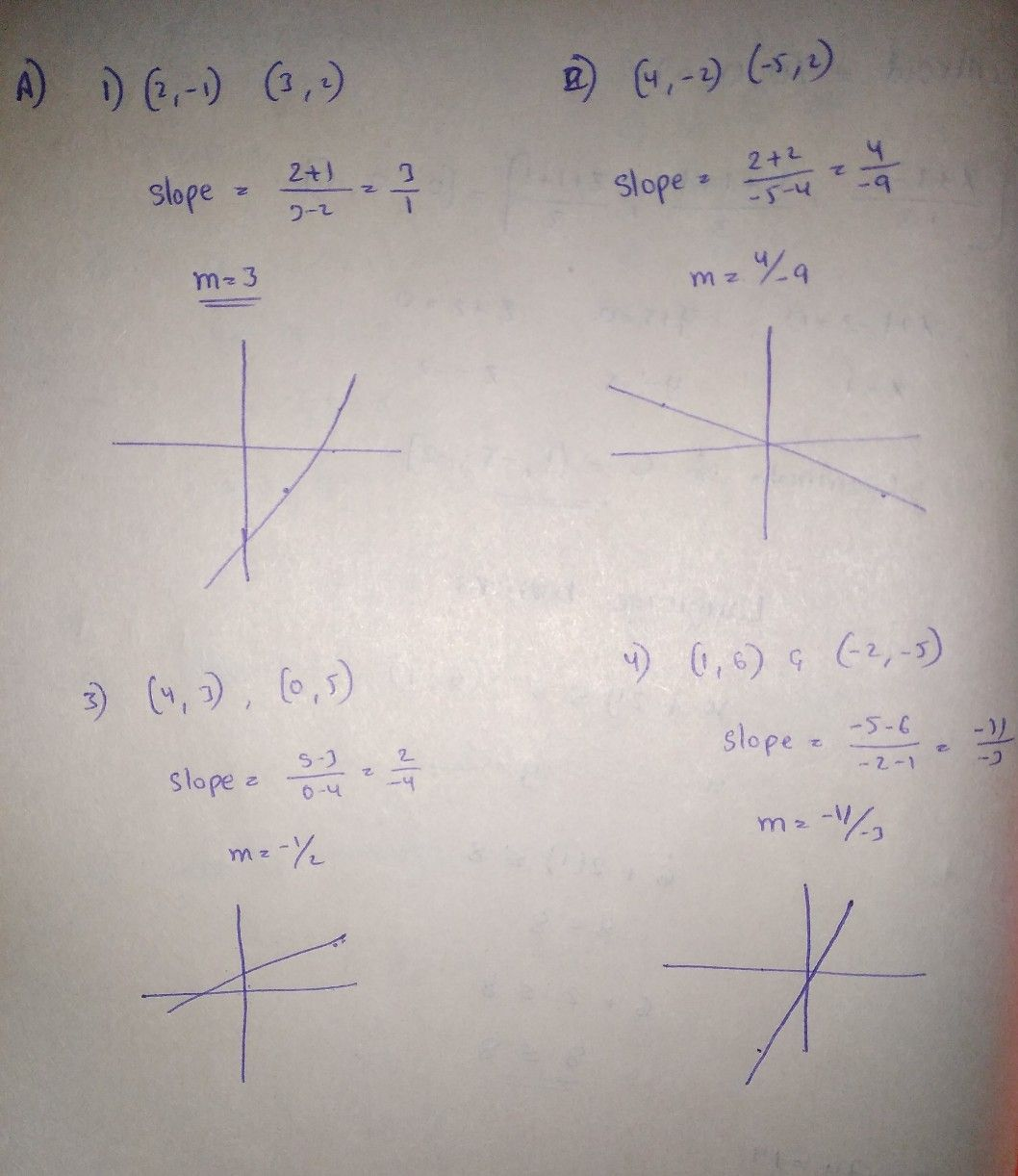Symbol
ProblemA. Using two points. 1. $\left(2,-1\right)$ and $\left(3,2\right)$ $3.$ $\left(4,3\right)$ and (0, 5) 2. $\left(4,-2\right)$ and $\left(-5,2\right)$ 4. $\left(1,6\right)$ and $\left(-2,-5\right)$ Find the slope of each line. Describe.the graph. B. Using intercepts 5. $\left(0,-3\right)$ and (1, 0) 7. (5, 0) and (0, -4) 6. (-3, 0) and $\left(0,-3\right)$ 8. (2. 0) and $\left(0,0$ -4) Find the slope of the line. Describe the graph. C. Using slope and a point 9. $m=5$ and $\left(6,-1\right)$ $11.m=-2$ and $\left(4,1\right)$ qand $\left(-3,-3\right)$ 10. m $\dfrac {3} {5}^{and}$ (2, -4) $12.m=$ $-\dfrac {4} {3}^{an}$
7th-9th grade
Other
Search count: 112
SolutionQanda teacher - Aravind